Lemma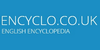In grasses, the lower bract that with the palea encloses the flower.

Lemma• (n.) A preliminary or auxiliary proposition demonstrated or accepted for immediate use in the demonstration of some other proposition, as in mathematics or logic.
Found on http://thinkexist.com/dictionary/meaning/lemma/

lemma(from the article `Poaceae`) ...closely overlapping scales. There are three kinds of scales. The lowermost, called glumes, are usually two in number, and they enclose some or all ...
Found on http://www.britannica.com/eb/a-z/l/33

Lemma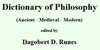(Gr. lemma) In Aristotle's logic a premiss of a syllogism. -- G.R.M. In mathematics, a theorem proved for the sake of its use in proving another theorem. The name is applied especially in cases where the lemma ceases to be of interest in itself after proof of the theorem for the sake of which it was introduced. -- A.C.
Found on http://www.ditext.com/runes/l.html

lemmanoun a subsidiary proposition that is assumed to be true in order to prove another proposition
Found on https://www.encyclo.co.uk/local/20974

Lemma[botany] Lemma is a phytomorphological term used in botany referring to a part of the spikelet of grasses (Poaceae). It is the lowermost of two chaff-like bracts enclosing the grass floret. It often bears a long bristle called an awn, and may be similar in form to the glumes - chaffy bracts at the base of each spikelet. It is usually interp...
Found on http://en.wikipedia.org/wiki/Lemma_(botany)

Lemma[logic] In informal logic and argument mapping, a lemma is simultaneously a contention for premises below it and a premise for a contention above it. ...
Found on http://en.wikipedia.org/wiki/Lemma_(logic)

Lemma[mathematics] In mathematics, a lemma (plural lemmata or lemmas) from the Greek λῆμμα (lemma, `anything which is received, such as a gift, profit, or a bribe”) or helping theorem is a proven proposition which is used as a stepping stone to a larger result rather than as a statement of interest by itself. There is no formal distincti...
Found on http://en.wikipedia.org/wiki/Lemma_(mathematics)

LemmaLem'ma (lĕm'mȧ) noun ; plural Latin Lemmata (-mȧ*tȧ), English Lemmas (-mȧz). [ Latin lemma , Greek lh^mma anything received, an assumption or premise taken for granted, from lamba`nein to take, assume. Confer Syll...
Found on http://www.encyclo.co.uk/webster/L/28

Lemma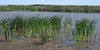A fertile scale found in grasses.
Found on http://www.encyclo.co.uk/local/22037

LemmaA fertile scale found in grasses.
Found on http://www.encyclo.co.uk/local/22038

LemmaA proposition that is useful mainly for the proof of some other theorem. See also: Theorem.
Found on http://www.diracdelta.co.uk/science/source/l/e/lemma/source.html

lemmaA short auxiliary proposition used in the proof of a larger theorem.
Found on http://www.daviddarling.info/encyclopedia/L/lemma.html

Lemma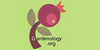In grasses, the flowering glume,
Found on http://www.gardenology.org/wiki/Glossary_of_Gardening_Terms

Lemma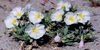in grasses, the lower and usually larger of the two bracts of the floret
Found on http://www.calflora.net/botanicalnames/botanicalterms.html

Lemma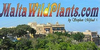in grasses, the lower and usually larger of the two bracts of the floret
Found on http://www.encyclo.co.uk/local/21767

Lemma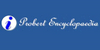In mathematics, a lemma is a preliminary proposition, laid down in order to clear the way for some following demonstration, and prefixed either to theorems, in order to render their demonstration less perplexed and intricate, or to problems, to make their solution easier and short.
Found on http://www.probertencyclopaedia.com/browse/GL.HTM

lemmaLatin, meaning: theme, title, epigram.
Found on http://archives.nd.edu/lll.htm

lemma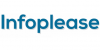lemma (lem'u) : see theorem.

lemma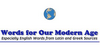lemma 1. In botany: The outer or lower of two bracts surrounding, or enclosing, the flower of a grass spikelet [small spike]. 2. In logic, a proposition that is assumed to be true in order to test the validity of another proposition. 3. An assumption taken for granted; something received; assumption; premise. 4. In publishing, a heading that indi...
Found on http://www.wordinfo.info/words/index/info/view_unit/1162/

lemma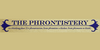preliminary proposition, theme, argument or headword
Found on http://phrontistery.info/l.html

Lemmathe dictionary form or gloss heading of an ancient Greek word.
Found on http://www.hestories.info/greco-roman-world-glossary.html

lemma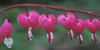the lower of 2 bracts enclosing a grass flower.
Found on http://en.wikipedia.org/wiki/Glossary_of_botanical_terms

lemmaThe lower of two bracts enclosing a grass flower. ... (09 Oct 1997) ...
Found on http://www.encyclo.co.uk/local/20973

lemma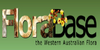the lower of two bracts of a grass floret, usually enclosing the palea, lodicules, stamens and ovary
Found on http://florabase.dpaw.wa.gov.au/help/glossary
No exact match found